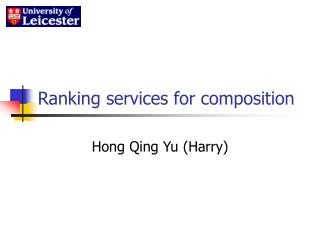DownloadDownload PresentationRanking services for composition

# Ranking services for composition

Télécharger la présentation## Ranking services for composition

- - - - - - - - - - - - - - - - - - - - - - - - - - - E N D - - - - - - - - - - - - - - - - - - - - - - - - - - -
##### Presentation Transcript

1. Ranking services for composition Hong Qing Yu (Harry)

2. Service composition “Composition of Web services has received much interest to support business-to-business or enterprise application integration.”  • Static • Dynamic 

3. Issues for composition • Global services registration • Service search/discovery • Understanding composition requirements • Service selection • Workflow generation • Service invoking

4. Ranking problem for selection • If there are more than two services satisfying functional requirements, • Which one is best to use? Cheapest one Fastest one Best performance • Other non-functional properties. • Logic Scoring preferenceis a technique can help us.

5. Logic scoring preference • Traditional Scoring Techniques are simple E=W1E1+W2E2+…+WnEn, 0 ≤ E ≤ 1. • There is a problem  It is regardless of the level of importance, the contribution of component Ei to the global score is limited to Wi • LSP (Logic Scoring preference)

6. Logic scoring preference • Differences are r & W E=(W1Er1+W2Er2+…+WnErn)1/r, 0 ≤ E ≤ 1, W1+W2+…+Wn=1, Wi>0, i=1,2,…,n. • r is a real number selected to achieve the desired logical properties of the aggregation function

7. Logic scoring preference  

8. Ranking by composition context : is an European project • The meaning of context in the project • Context affects service selection • We need a simpler way to define r

9. Designing evaluation rules E=(W1Er1+W2Er2+…+WnErn)1/r, 0 ≤ E ≤ 1, W1+W2+…+Wn=1, Wi>0, i=1,2,…,n. • Filtering rules • Evaluation function • r selection

10. Filtering rules Quality>85 Cost<\$35 Speed>30/s Irreplaceable preference criteria Replaceable preference criteria If the service’s properties do not achieve the irreplaceable preference, then it will be filtered out.

11. Evaluation function • Exact match Es=1 (if the criteria is matched) or 0 (if is not matched) • Set overlap Es=(e1+e2+…+ei) /i (with Ei being a score for each criteria) • Level match if i is the number of levels and ic is current service level value, then we define: Es=ic/i

12. Evaluation function • Specific value if vx is the maximum value of all relevant services in one criteria, vn is the minimum value and vi is the current service value, then we calculate:

13. r selection • E=W1E1+W2E2+...WnEn • Can we compute the weight for choosing the r instead of using the way introduced in . • On the one hand, Filter makes all aspects criteria is replaceable, which means that we need conjunction. • On the other hand, if the weight of each criterion are so difference, we also need disjunction.

14. r selection rules • We are in a very balanced position, and we can narrow our r selection tables • To simplify defining the r value, we just select 1.5, 1, 0.5. • If (highest weight – lowest weight)>average weight, then r=1.5 • If (highest weight – lowest weight)<average weight, then r=0.5 • If (highest weight – lowest weight)=average weight, then r=1

15. Example

16. Worked Example • Criterion requirement: • More people’s weight=0.6 • Quality’s weight=0.3 • Cost’s weight=-0.1 • The result Eskype=(2/3)1.5·0.6+(2/3)1.5·0.3+11.5·0.1=0.590 Etalkfly=11.5·0.6 +(1/3)1.5·0.3+0=0.658 Ehotmail =11.5·0.6+11.5·0.3+(0.6)1.5·0.1=0.946

17. References • http://www.zurich.ibm.com/pdf/ebizz/icaps-ws.pdf • http://www.active-endpoints.com/open-source-tutorial.htm • http://www.isi.edu/~thakkar/icaps2003-p4ws.pdf • http://citeseer.ist.psu.edu/cache/papers/cs/2874/http:zSzzSzcs.sfsu.eduzSzpeoplezSzjozozSzlsp.pdf/a-method-for-evaluation.pdf • “Continuous Preference Logic for System Evaluation”, Jozo J. Dujmovic, USA

18. Thanks Questions